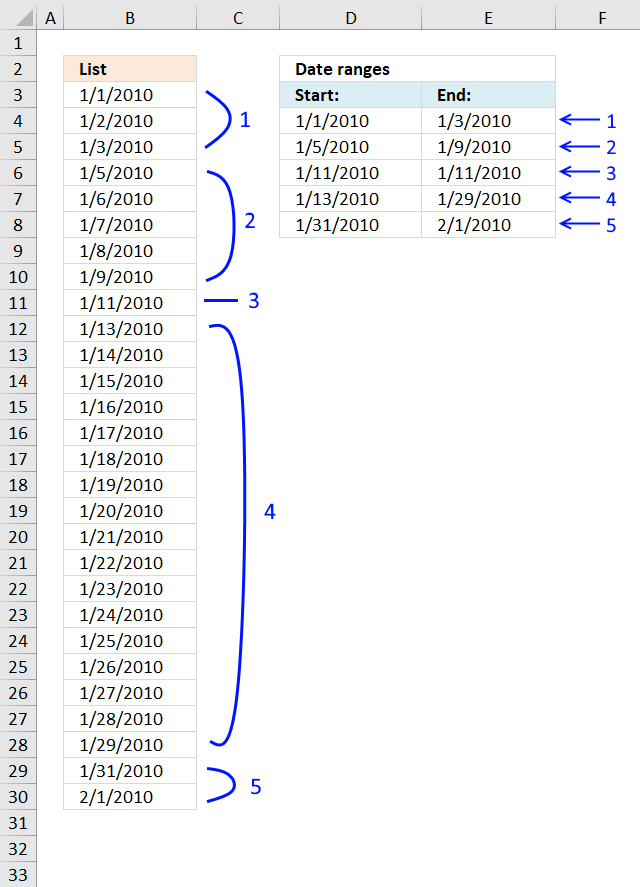Author: Oscar Cronquist Article last updated on December 23, 2018The array formula in cell D4 extracts the start dates for date ranges in cell range B3:B30, the array formula in cell E4 extracts the end dates for date ranges in cell range B3:B30.

The dates in column B must be sorted from smallest to largest for the formula to work properly, the image above shows the date ranges (blue lines) and the numbers correspond to the extracted date ranges displayed in column D and E.

Array formula in cell D4:

=IF(ROWS(\$B\$2:B2)=1, INDEX(\$B\$3:\$B\$30, 1), INDEX(\$B\$3:\$B\$30, SMALL(IF(\$B\$4:\$B\$30-\$B\$3:\$B\$29>1, ROW(\$B\$3:\$B\$29)-MIN(ROW(\$B\$3:\$B\$29))+2, ""), ROWS(\$B\$1:B1)-1)))

How to create an array formula

1. Select cell C2
2. Paste formula
3. Press and hold CTRL + SHIFT
4. Press Enter

Copy cell C2 and paste it down as far as needed.

Array formula in cell E4:

=IF(SUMPRODUCT((\$B\$4:\$B\$30-\$B\$3:\$B\$29>1)*1)=ROW(A1)-1, INDEX(\$B\$3:\$B\$30, COUNT(\$B\$3:\$B\$30)), INDEX(\$B\$3:\$B\$30, SMALL(IF(\$B\$4:\$B\$30-\$B\$3:\$B\$29>1, ROW(\$B\$3:\$B\$29)-MIN(ROW(\$B\$3:\$B\$29))+1, ""), ROW(A1))))

Copy cell D2 and paste it down as far as needed.

### Explaining formula in cell D5

The formula extracts the first date if the current cell is the first cell that is why I start with formula in cell D5.

The ROWS function returns the number of rows in a cell reference, when the cell is copied to cells below the cell reference grows, this is how the formula keeps track of where the first cell is.

IF(ROWS(\$B\$2:B2)=1,INDEX(\$B\$3:\$B\$30,1), formula)

ROWS(\$B\$2:B2)=1

becomes

1=1 equals TRUE.

IF(TRUE,INDEX(\$B\$3:\$B\$30,1), formula)

becomes

INDEX(\$B\$3:\$B\$30,1)

and returns the first date in cell \$B\$3:\$B\$30 (1/1/2010).

#### Step 1 - Compare dates

If we subtract a date with the next date in a sorted list we can quickly check if the next date is one day after the first date. If the number is larger than 1 we know the first date must be an end date and the next date must be a start date.

\$B\$4:\$B\$30-\$B\$3:\$B\$29>1

becomes

{40180; 40181; 40183; 40184; 40185; 40186; 40187; 40189; 40191; 40192; 40193; 40194; 40195; 40196; 40197; 40198; 40199; 40200; 40201; 40202; 40203; 40204; 40205; 40206; 40207; 40209; 40210} - {40179; 40180; 40181; 40183; 40184; 40185; 40186; 40187; 40189; 40191; 40192; 40193; 40194; 40195; 40196; 40197; 40198; 40199; 40200; 40201; 40202; 40203; 40204; 40205; 40206; 40207; 40209}>1

becomes

{1; 1; 2; 1; 1; 1; 1; 2; 2; 1; 1; 1; 1; 1; 1; 1; 1; 1; 1; 1; 1; 1; 1; 1; 1; 2; 1}>1

and returns

{FALSE; FALSE; TRUE; FALSE; FALSE; FALSE; FALSE; TRUE; TRUE; FALSE; FALSE; FALSE; FALSE; FALSE; FALSE; FALSE; FALSE; FALSE; FALSE; FALSE; FALSE; FALSE; FALSE; FALSE; FALSE; TRUE; FALSE}

#### Step 2 - Replace TRUE with corresponding row number

The IF function has three arguments, the first one must be a logical expression. If the expression evaluates to TRUE then one thing happens (argument 2) and if FALSE another thing happens (argument 3).

IF(\$B\$4:\$B\$30-\$B\$3:\$B\$29>1,ROW(\$B\$3:\$B\$29)-MIN(ROW(\$B\$3:\$B\$29))+2,"")

becomes

IF({FALSE; FALSE; TRUE; FALSE; FALSE; FALSE; FALSE; TRUE; TRUE; FALSE; FALSE; FALSE; FALSE; FALSE; FALSE; FALSE; FALSE; FALSE; FALSE; FALSE; FALSE; FALSE; FALSE; FALSE; FALSE; TRUE; FALSE},ROW(\$B\$3:\$B\$29)-MIN(ROW(\$B\$3:\$B\$29))+2,"")

becomes

IF({FALSE; FALSE; TRUE; FALSE; FALSE; FALSE; FALSE; TRUE; TRUE; FALSE; FALSE; FALSE; FALSE; FALSE; FALSE; FALSE; FALSE; FALSE; FALSE; FALSE; FALSE; FALSE; FALSE; FALSE; FALSE; TRUE; FALSE},{2; 3; 4; 5; 6; 7; 8; 9; 10; 11; 12; 13; 14; 15; 16; 17; 18; 19; 20; 21; 22; 23; 24; 25; 26; 27; 28},"")

and returns

{""; ""; 4; ""; ""; ""; ""; 9; 10; ""; ""; ""; ""; ""; ""; ""; ""; ""; ""; ""; ""; ""; ""; ""; ""; 27; ""}.

#### Step 3 - Extract k-th smallest row number

The SMALL function lets you calculate the k-th smallest value in a cell range or array. SMALL( arrayk). This makes sure that a new date is displayed in each cell below. The k argument contains an expanding cell reference that grows when the cell is copied to cells below.

SMALL(IF(\$B\$4:\$B\$30-\$B\$3:\$B\$29>1, ROW(\$B\$3:\$B\$29)-MIN(ROW(\$B\$3:\$B\$29))+2, ""), ROWS(\$B\$1:B2)-1)

becomes

SMALL({""; ""; 4; ""; ""; ""; ""; 9; 10; ""; ""; ""; ""; ""; ""; ""; ""; ""; ""; ""; ""; ""; ""; ""; ""; 27; ""}, ROWS(\$B\$1:B2)-1)

becomes

SMALL({""; ""; 4; ""; ""; ""; ""; 9; 10; ""; ""; ""; ""; ""; ""; ""; ""; ""; ""; ""; ""; ""; ""; ""; ""; 27; ""}, 1)

and returns 4.

#### Step 4 - Return value

The INDEX function returns a value based on a cell reference and column/row numbers.

INDEX(\$B\$3:\$B\$30,SMALL(IF(\$B\$4:\$B\$30-\$B\$3:\$B\$29>1,ROW(\$B\$3:\$B\$29)-MIN(ROW(\$B\$3:\$B\$29))+2,""),ROWS(\$B\$1:B1)-1))

becomes

INDEX(\$B\$3:\$B\$30, 4)

and returns 1/5/2010 in cell D5.